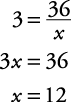## Variations

A variation is a relation between a set of values of one variable and a set of values of other variables.

#### Direct variation

In the equation y = mx + b, if m is a nonzero constant and b = 0, then you have the function y = mx (often written y = kx), which is called a direct variation. That is, you can say that y varies directly as x or y is directly proportional to x. In this function, m (or k) is called the constant of proportionality or the constant of variation. The graph of every direct variation passes through the origin.

##### Example 1

Graph y = 2 x.x

y

0

0

1

2

2

4

##### Example 2

If y varies directly as x, find the constant of variation when y is 2 and x is 4.

Because this is a direct variation,

y = kx (or y = mx)

Now, replacing y with 2 and x with 4,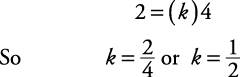The constant of variation is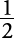.

##### Example 3

If y varies directly as x and the constant of variation is 2, find y when x is 6.

Since this is a direct variation, simply replace k with 2 and x with 6 in the following equation.A direct variation can also be written as a proportion.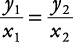This proportion is read, “ y 1 is to x 1 as y 2 is to x 2.” x 1 and y 2 are called the means, and y 1 and x 2 are called the extremes. The product of the means is always equal to the product of the extremes. You can solve a proportion by simply multiplying the means and extremes and then solving as usual.

##### Example 4

r varies directly as p. If r is 3 when p is 7, find p when r is 9.

Method 1. Using proportions: Set up the direct variation proportion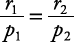Now, substitute in the values.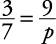Multiply the means and extremes (cross multiplying) give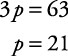Method 2. Using y = kx:

Replace the y with p and the x with r.

p = kr

Use the first set of information and substitute 3 for r and 7 for p, then find k.Rewrite the direct variation equation as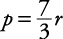.

Now use the second set of information that says r is 9, substitute this into the preceding equation, and solve for p.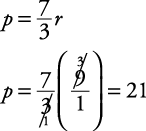#### Inverse variation (indirect variation)

A variation where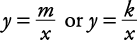is called an inverse variation (or indirect variation). That is, as x increases, y decreases. And as y increases, x decreases. You may see the equation xy = k representing an inverse variation, but this is simply a rearrangement of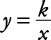.

This function is also referred to as an inverse or indirect proportion. Again, m (or k) is called the constant of variation.

##### Example 5

If y varies indirectly as x, find the constant of variation when y is 2 and x is 4.

Since this is an indirect or inverse variation,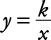Now, replacing y with 2 and x with 4,The constant of variation is 8.

##### Example 6

If y varies indirectly as x and the constant of variation is 2, find y when x is 6.

Since this is an indirect variation, simply replace k with 2 and x with 6 in the following equation.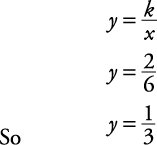As in direct variation, inverse variation also can be written as a proportion.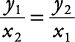Notice that in the inverse proportion, the x 1 and the x 2 switched their positions from the direct variation proportion.

##### Example 7

If y varies indirectly as x and y = 4 when x = 9, find x when y = 3.

Method 1. Using proportions: Set up the indirect variation proportion.Now, substitute in the values.Multiply the means and extremes (cross‐multiplying) givesMethod 2. Using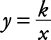: Use the first set of information and substitute 4 for y and 9 for x, then find k.Rewrite the direct variation equation as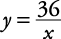.

Now use the second set of information that says y is 3, substitute this into the preceding equation and solve for x.